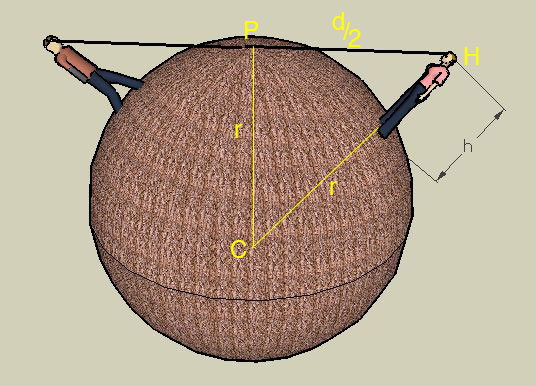SEARCH HOMEMath Central Quandaries & QueriesQuestion from Ben, a student: Two people who are both h feet tall are standing on a spherical planet. One person walks a distance d in feet away from the the other person. At this point, the person walking turns around and can no longer see the top of the other persons head. What is the radius of the planet?Hi Ben,

Here is our diagram. C is the centre of the plante, P is the point where the line of sight is tangent to the planet surface, H is the top of the head of one of the people and r is the radius of the planet.Since triangle HPC is a right triangle Pythagoras' Theorem tell us

r2 + (d/2)2 = (r + h)2

Solve for r.

Seephen La Rocque and Harley WestonMath Central is supported by the University of Regina and The Pacific Institute for the Mathematical Sciences.